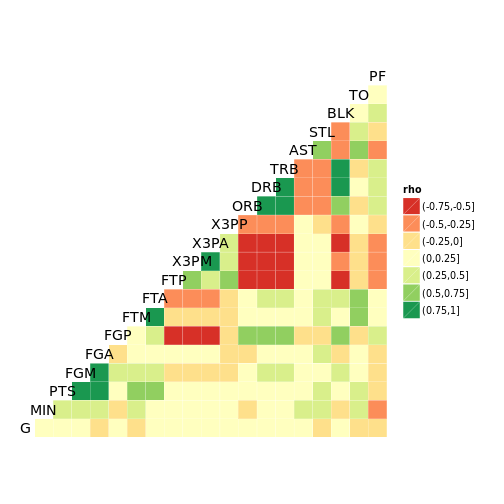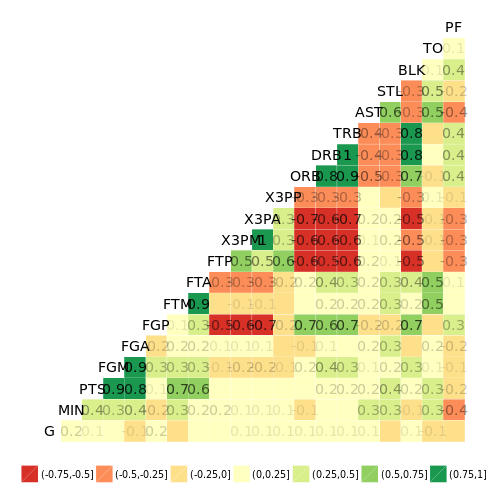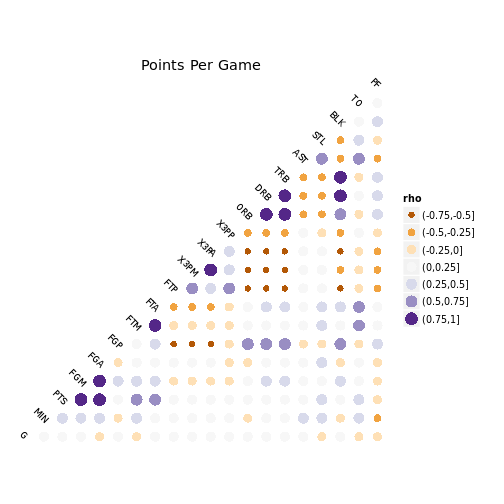# ggcorr - Plot a correlation matrix with ggplot2

``````# Basketball statistics provided by Nathan Yau at Flowing Data.
# Default output.
ggcorr(nba[, -1])````````````# Labelled output, with coefficient transparency.
ggcorr(nba[, -1],
label = TRUE,
label_alpha = TRUE,
name = "") +
ggplot2::theme(legend.position = "bottom")````````````# Custom options.
ggcorr(
nba[, -1],
geom = "circle",
max_size = 6,
size = 3,
hjust = 0.75,
angle = -45,
palette = "PuOr" # colorblind safe, photocopy-able
) + ggplot2::labs(title = "Points Per Game")``````
``## Warning: Removed 210 rows containing missing values (geom_point).``Updated